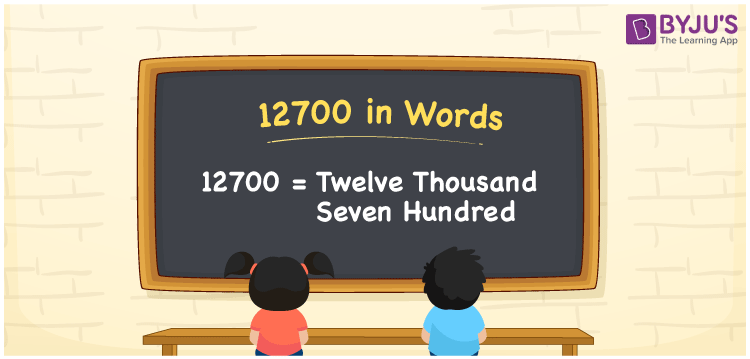# 12700 in Words

12700 in words is written as “Twelve thousand seven hundred”. For example, Rupees 12700 is mentioned as “Rupees Twelve thousand seven hundred only” in a cheque. In Mathematics, 12700 is a cardinal number that is used to represent the quantity or value of something. The name of 12700 in English is Twelve thousand seven hundred. Learn to write the number 12700 in words using the place value method in this article.

 12700 in words Twelve thousand seven hundred Twelve thousand seven hundred in numerical form 12700

## 12700 in English Words## How to Write 12700 in words?

12700 is a five-digit number that can be written in words, by knowing the positions of each digit. The place value of each digit in 12700 is given in the below table.

 Ten Thousands Thousands Hundreds Tens Ones 1 2 7 0 0

We can see, from the above table,

1 → Ten thousand

2 → Thousand

7 → Hundred

0 → Tens

0 → Ones

Hence, when we go from right to left in the table, the number 12700 is read as Twelve thousand seven hundred. Learn more about Numbers In Words at BYJU’S.

### Expanded Form of 12700

We can write the expanded form as:

1 × Ten thousand + 2 × Thousand + 7 × Hundred + 0 × Ten + 0 × One

= 1 × 10000 + 2 × 1000 + 7 × 100 + 0 × 10 + 0 × 1

= 12700

= Twelve thousand seven hundred

12700 is the natural number that is succeeded by 12699 and preceded by 12701. Learn more about the number 12700 below:

• 12700 in words – Twelve thousand seven hundred
• Is 12700 an odd number? – No
• Is 12700 an even number? – Yes
• Is 12700 a perfect square number? – No
• Is 12700 a perfect cube number? – No
• Is 12700 a prime number? – No
• Is 12700 a composite number? – Yes

## Frequently Asked Questions on 12700 in words

Q1

### What is 12700 in words?

12700 in words is written as Twelve thousand seven hundred.
Q2

### What is the place value of 7 in 12700?

The place value of 7  in 12700 is Hundreds.
Q3

### How to write 12700 in English words?

12700 in English is spelt as Twelve thousand seven hundred.
Test your Knowledge on 12700 in Words